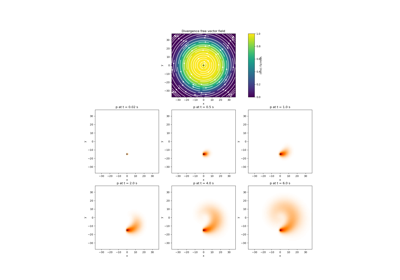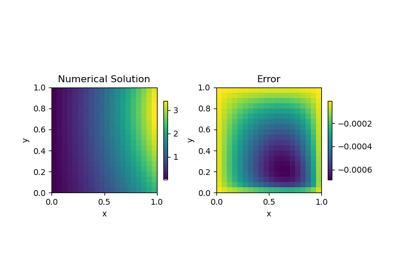# discretize.mixins.InterfaceMPL#class discretize.mixins.InterfaceMPL[source]#

Class for plotting discretize meshes with matplotlib.

This interface adds three plotting methods to all discretize meshes. plot_grid will plot gridded points for 2D and 3D meshes. plot_image is used for plotting models, scalars and vectors defined on a given mesh. And plot_slice is used for plotting models, scalars and vectors on a 2D slice through a 3D mesh.

Methods

 plotGrid(*args, **kwargs) plotGrid has been deprecated. plotImage(*args, **kwargs) plotImage has been deprecated. plotSlice(*args, **kwargs) plotSlice has been deprecated. plot_3d_slicer(v[, xslice, yslice, zslice, ...]) Plot slices of a 3D volume, interactively (scroll wheel). plot_grid([ax, nodes, faces, centers, ...]) Plot the grid for nodal, cell-centered and staggered grids. plot_image(v[, v_type, grid, view, ax, ...]) Plot quantities defined on a given mesh. plot_slice(v[, v_type, normal, ind, ...]) Plot a slice of fields on the given 3D mesh.

## Galleries and Tutorials using discretize.mixins.InterfaceMPL#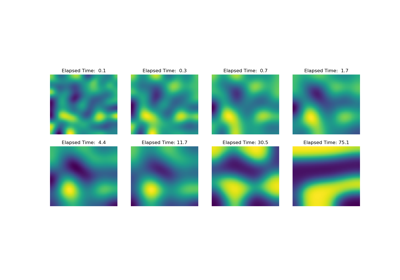Operators: Cahn Hilliard

Operators: Cahn Hilliard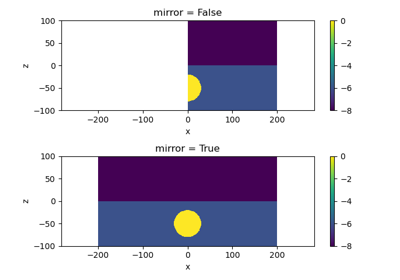Plot Mirrored Cylindrically Symmetric Model

Plot Mirrored Cylindrically Symmetric Model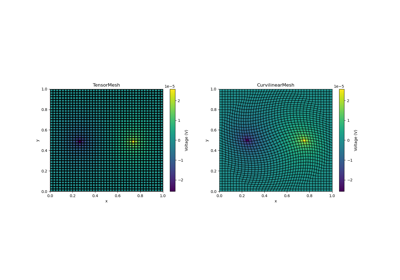Basic Forward 2D DC Resistivity

Basic Forward 2D DC ResistivityBasic: PlotImage

Basic: PlotImage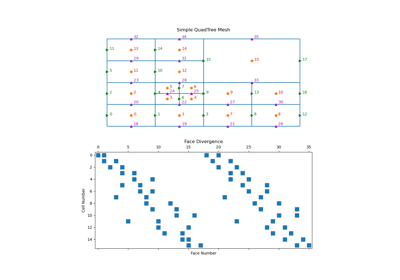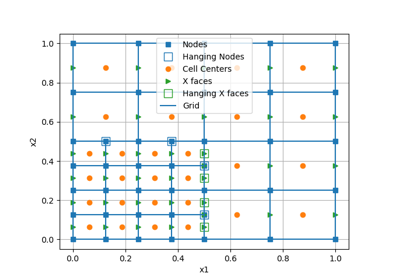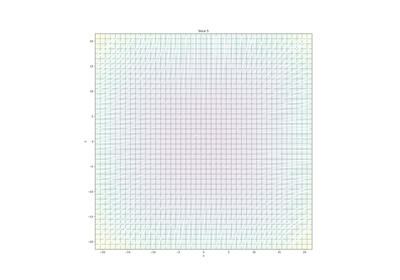Plotting: Streamline thickness

Plotting: Streamline thickness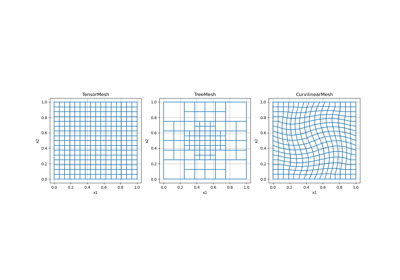Overview of Mesh Types

Overview of Mesh Types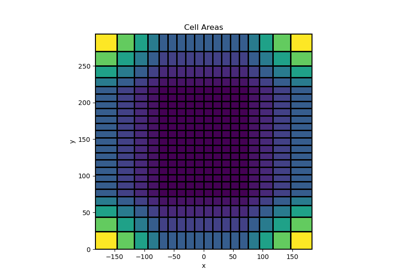Tensor meshes

Tensor meshes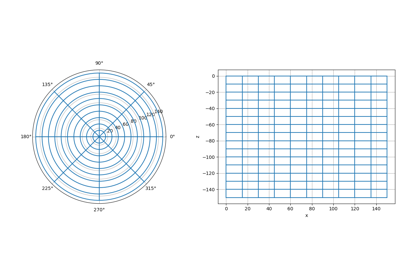Cylindrical meshes

Cylindrical meshes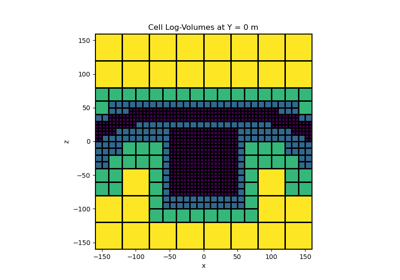Tree Meshes

Tree Meshes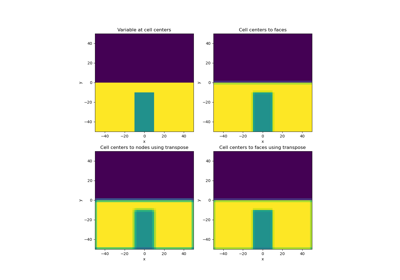Averaging Matricies

Averaging Matricies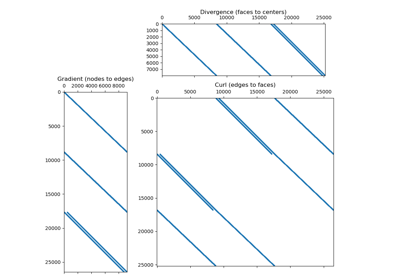Differential Operators

Differential Operators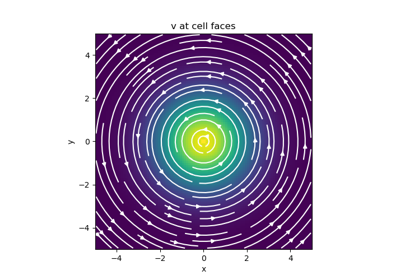Basic Inner Products

Basic Inner Products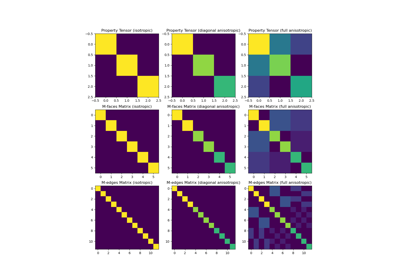Constitutive Relations

Constitutive Relations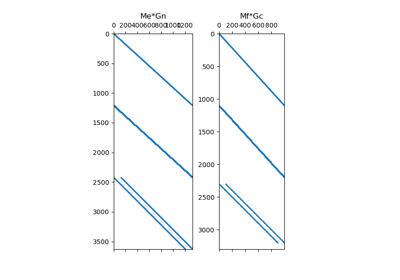Differential Operators

Differential Operators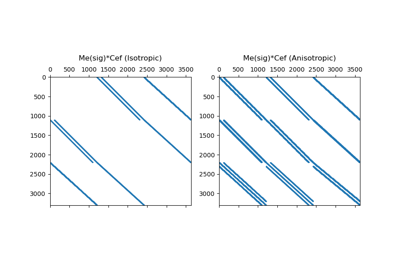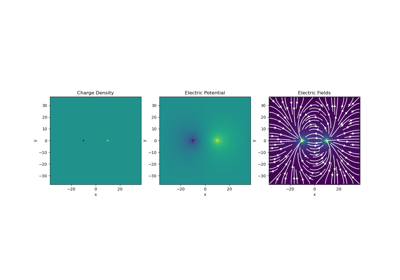Gauss’ Law of Electrostatics

Gauss' Law of Electrostatics